# Starting With ggplot2 in R

Harsh is a SQL DBA having experience of over 13 years. He has worked on most technologies related to SQL Server. He loves politics , reading

## Introducing ggplot2 Package

One of the most important aspects of data analytics is the visualization of the data. Visualization is probably the most powerful aspect that allows you view your data from different angles. It also allows you to put your conclusions across the board very powerfully. An image is worth a thousand words.

R has thousands of different packages that can do variety of tasks. ggplot2 is one such package which is designed for creating and displaying plots.

So in this article, I am going to show how we can construct a plot using ggplot2 in R from scratch. I am going to start with a blank plot and then add elements to it to build some basic plots.

Note that the package is named ggplot2.

The actual function that we will use for creating plots is named ggplot.

This can be confusing, but I am afraid that's how they are named.

So the package is ggplot2 and function that we use from that package is named ggplot.

Right.

## Objective

The objective of this article is not to build a fancy and amazing plot. The objective is to introduce the reader to the process of building a plot bit by bit from scratch.

Using this article, you should be able to understand different elements of ggplot2 plotting system and how to use them. However, please note that this is just a basic introduction of ggplot2 plotting system. In reality, ggplot2 is a very powerful but extremely vast plotting system and you can easily write a book on it.

However, this post will cover some basic building blocks of a ggplot graph and build three graphs using those basic building blocks.

## Building Blocks of ggplot

Before we do that, we need to understand the basic building blocks of a ggplot graph.

• Plot – This is the plotting area on which we will build the plot.
• Data – This is the data that will be used in the plot.
• Aesthetic mapping –This is the organization of your data on the plot. This tells ggplot which data points go on which axis, what color they should be, that shape they should be etc. Aesthetic mapping basically controls the visual aspect of the geometric objects that we plot.
• Geom – These are the different geometric objects that we will place on the plot area. They can be shapes like a dot for a scatter plot, lines, curves, etc. These objects represent your data on the plot.

Each of these blocks is represented by functions in R. So basically for each of these blocks, we will write a function.

Scroll to Continue

There is a lot more to ggplot than this, but for the time being we will start with actually seeing how these four elements work.

## Let's Get Started

So without any further ado, let’s fire up R and start building a ggplot graph.

But before you can start exploring ggplot2, you need to install it if you already haven’t done so.

## Install ggplot2 Package

Once this installation completed successfully, let’s load this package.

## Create a Blank Plot

Now that we have installed and loaded ggplot2 package, let’s build a plot from scratch. So first we need to build the first element we introduced earlier.

Plot – This is the plotting area on which we will build the plot.

That’s it. Note that the function name that we used is ggplot. It is not ggplot2. ggplot2 is the package name which contains this function.

This will create an empty plot. You should be able to see this in plots window of R Studio.

## Feed Data to Plot

Now, let’s move to the second point.

Data – This is the data that will be used in the plot.

Let’s give some data to ggplot. This will not be plotted. But we are just making some data accessible to the plot. Also please note that ggplot only accepts data frame object as the data. It will not accept a matrix, vector, list, or any other data type. I don’t understand this limitation but that’s how it is.

For this demonstration I am going to use an inbuilt data set in R named iris. This is part of the base R and you don’t need to install any additional package for this.

You can see what this data is by running the following command in R.

As you can see, it has five fields. Four of these fields are numeric and the last one is categorical. This data set is measurements of 150 flowers of 3 different species of IRIS flower.

This data set has four numeric measurements and one field identifying the species of the flower. Now, we will use this data set and see how we can plot this data using ggplot2.

Now, let’s feed this data to the ggplot. You do this by passing a parameter named data to ggplot function as shown below. The data that we fed to ggplot is a data frame named iris.

## Aesthetic Mapping

Your plot will still be blank. By this command, we have just passed the data frame iris to ggplot. Now let’s get to the third point.

Aesthetic mapping –This is the organization of your data on the plot.

Now we will define the aesthetic mapping for the data. In its simplest form, we just define what data needs to go on X axis and what needs to go on Y axis. You do this by passing another function named aes to ggplot function.

By this command, we have told ggplot to put Sepal Length on X axis and Sepal Width on Y axis. Now, let’s take a look at our plot. It looks like this.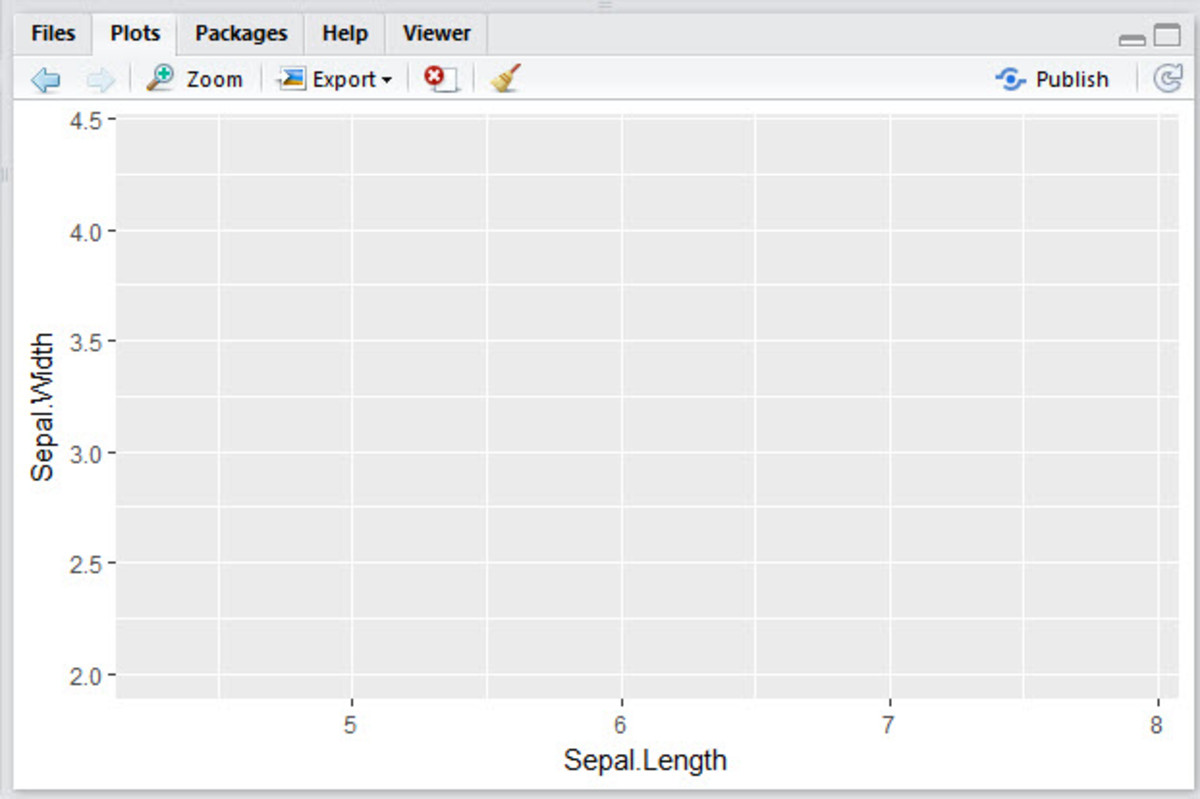Plot with X and Y axis mapping

Earlier the plot was blank. Now we can see two axes. On X axis we see Sepal Length and on Y axis we can see Sepal Width. It has also plotted a nice little grid based on values of Sepal Length and Sepal Width.

But we still don’t see any data points on the plot. All that our command has done is to format the plot. That’s exactly what ggplot function will do.

Now we will get to the fourth point.

## Geom

The actual plotting of data on the plot will be done by geometric objects, i.e., geom. Now, let’s add the geom to our plot.

For this we add geom._* functions to ggplot function as shown below. Note that this command is not complete. But when you type up to this point, you will see a list of geom options that are available to you.

You can see the options in the screen shot below. Which geom you choose depends on what kind of plot you want.

Now, let’s complete the command. For this demonstration, I will plot a scatter plot which is just points.

Now, let’s take a look at our plot.

## Our First Plot With ggplot

And there you are. Your first plot with ggplot is ready.

But it’s a bit dull, isn’t it? Let’s add some color to it.

Can you spot the difference between this command and the earlier one? I added a parameter called color to geom point and passed it a value of red. This tells ggplot to color all the points red.

This is how our plot looks now.

Well, let’s say I am bored of dots in my scatter plot and I want to change the shape of my points. I add one more parameter named shape and pass it value of four. As you can see in the screenshot below this command, ggplot has changed shape of the points in the scatter plot.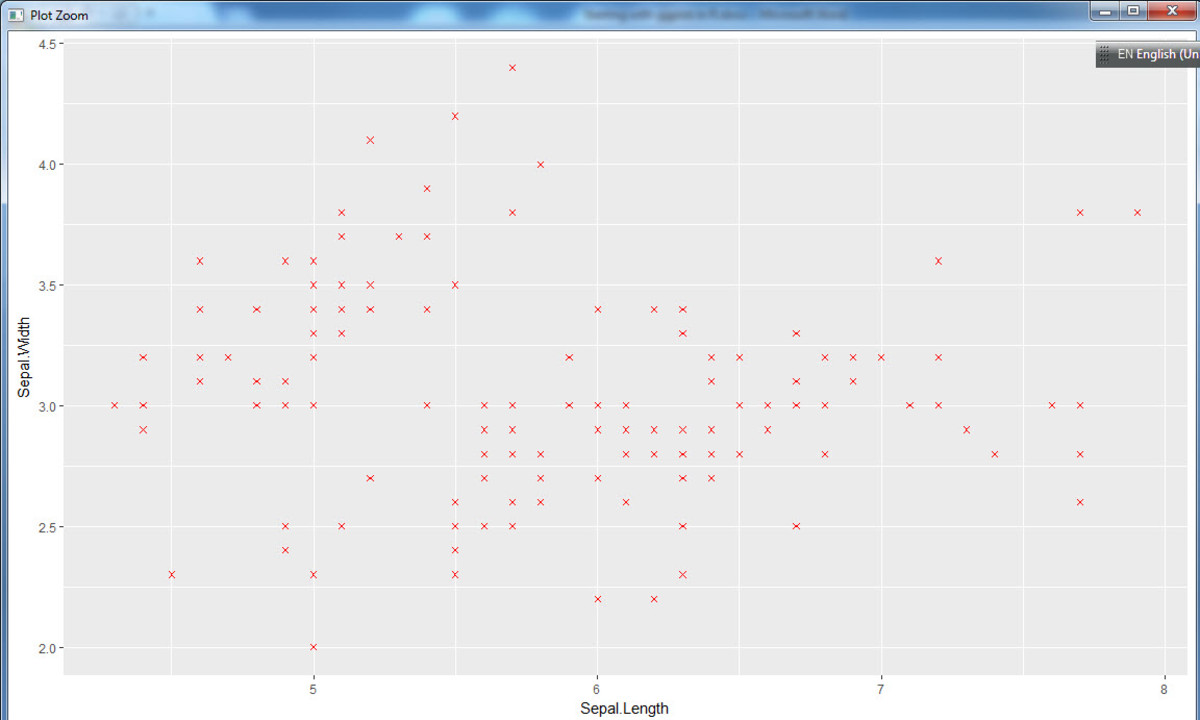Scatter plot in red but with cross instead of dots

Well, I suppose you get the picture, don’t you? To change the points, you add more parameters to the geom function.

What parameters you can pass depends on the geom you are using. This is just the tip of the iceberg and if you start digging deeper in ggplot, you would find the opportunities almost endless.

Now, let’s change the geom from point to a line. This will generate a line plot instead of scatter plot.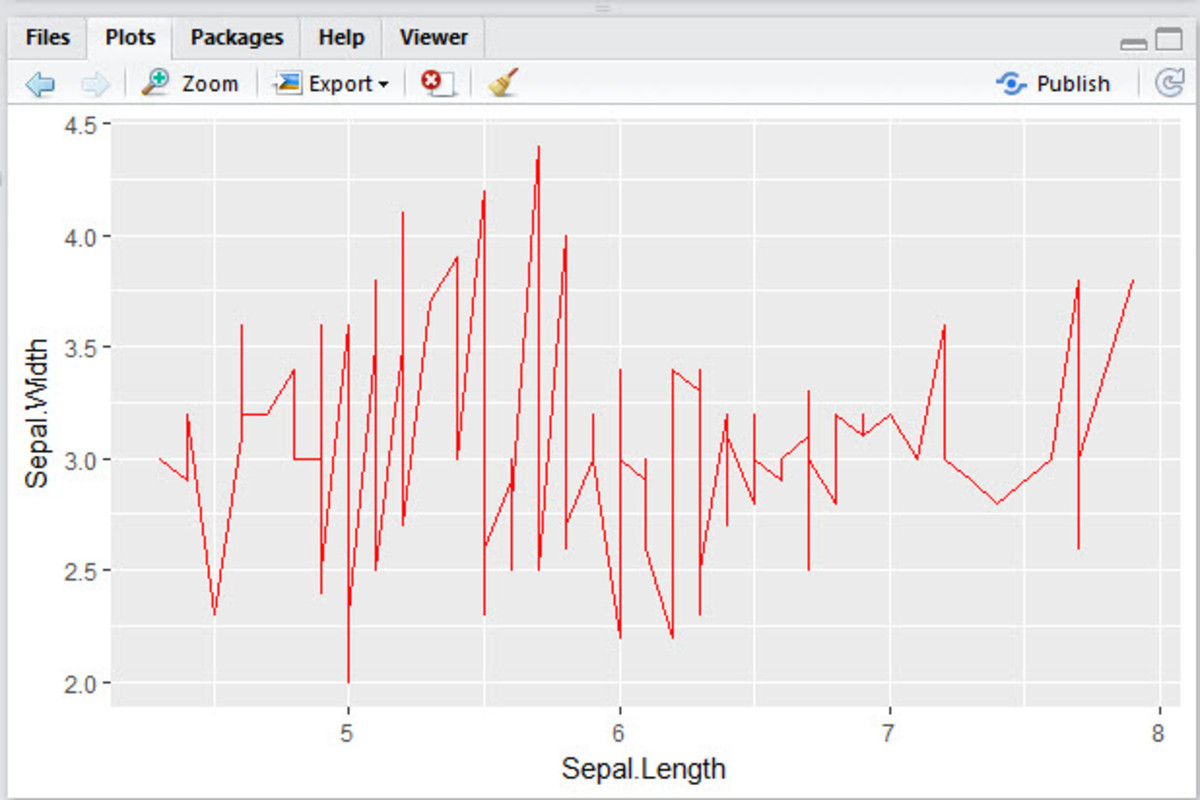Line plot instead of scatter plot. Looks a bit ugly, doesn't it?

As you can see it changed the points to line. How about plotting one numeric variable and other categorical one?

In our data iris, species is a categorical data. It is not numeric like length or width but a class.

Let’s plot another scatter plot but instead of Sepal Length on X axis, let’s plot Species on X axis. You can see that for this, I have to change ggplot function aes. Instead of Sepal Length I have passed Species to x axis.

And this is the output we get.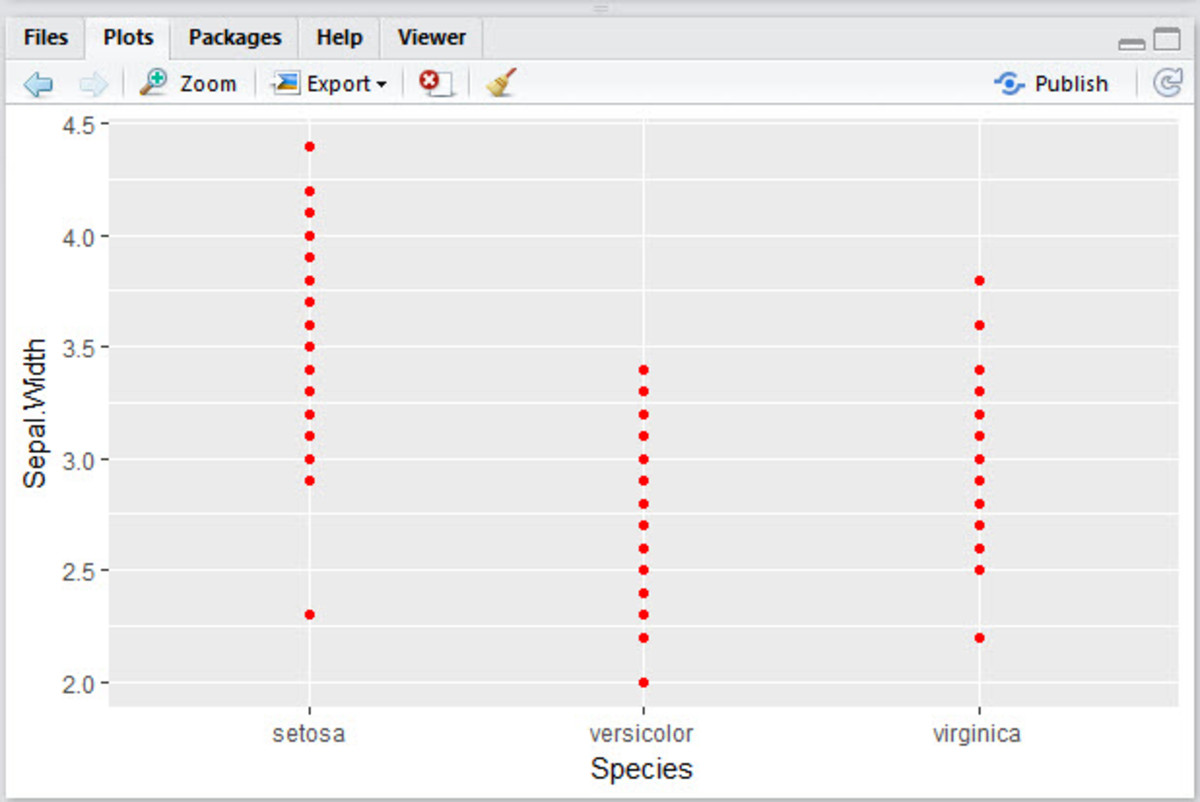Scatter plot with one numeric variable and one categorical variable

But generally, if you want to plot one categorical variable against a numeric variable, you might want to plot a box plot instead of scatter plot. Box plot shows median, minimum, maximum values and it also shows outliers.

So now let’s plot box plot instead of scatter plot. So now we change the geom from point to box plot.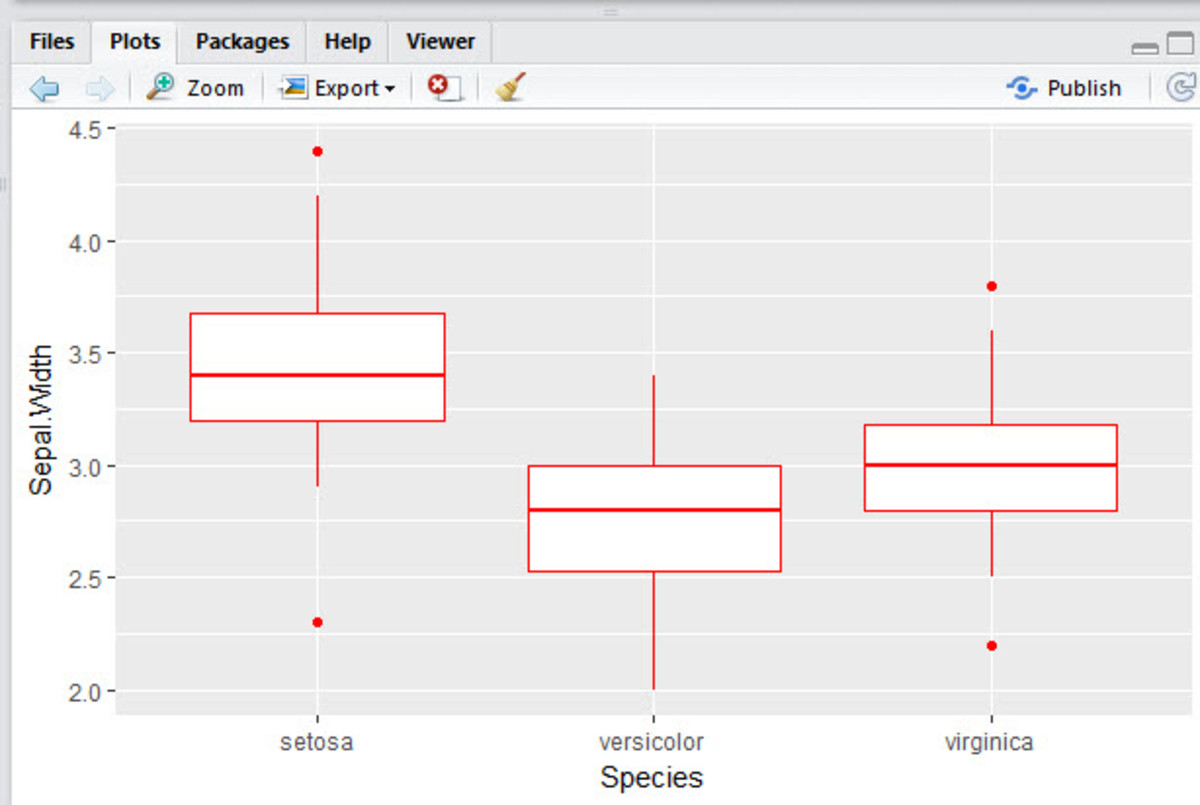Box plot between a numeric and categorical variable

Are you bored of red yet? Let’s change the color of these boxes and also add a fill color inside the boxes.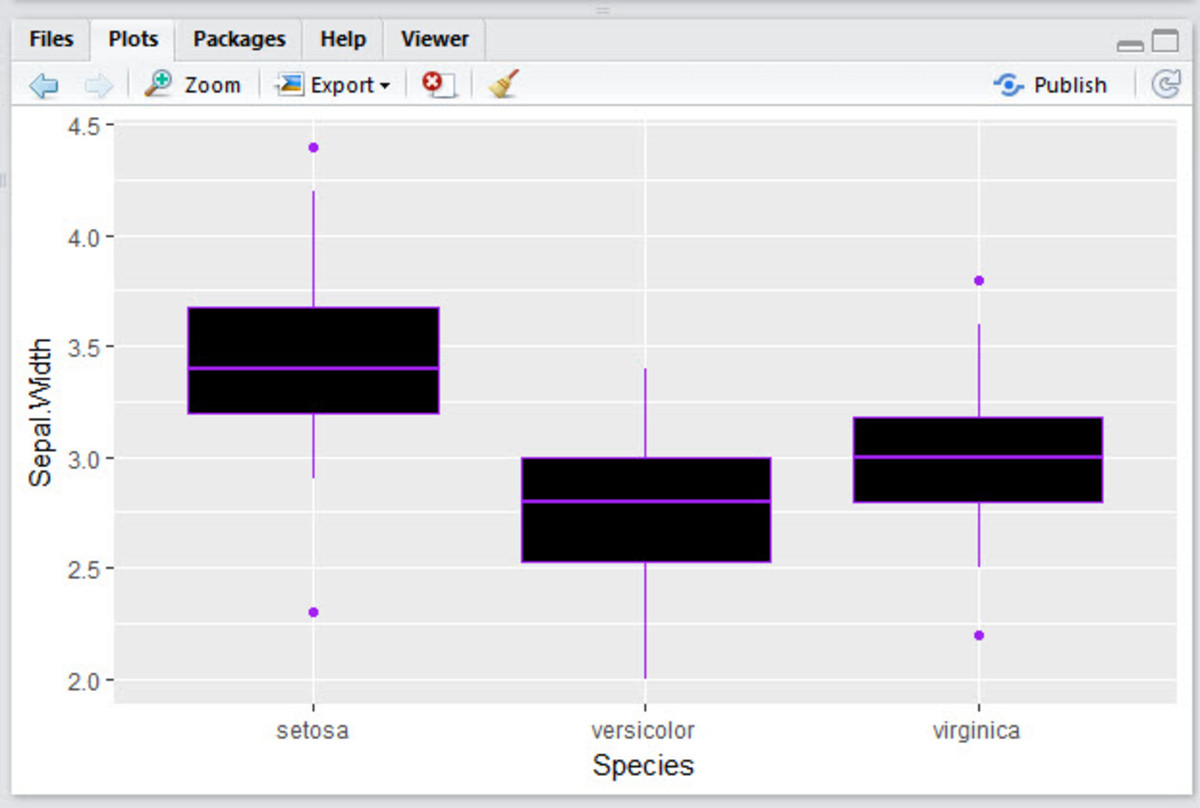Box plot with color and fill

## Summary

So till now, we have created a scatter plot, line plot and a box plot. We have added some color to it.

You can do a lot more than these 3 plots that I illustrated till now. Realistically speaking the power of ggplot is almost amazing.

This is one handy tool to have in your toolbox.

## Limitations

But just like any tool, it also has its limitations. It certainly can’t do some stuff that lattice can do. It’s not very good with 3D plots and you may need to use rgl for that. It can’t handle graph theory type graphs that have nodes or decision tree structures.

So that’s it for this time folks. Please let me know what you think in the comments section below. If you want any improvements in this post please let me know and I would be glad to implement our suggestions.

This article is accurate and true to the best of the author’s knowledge. Content is for informational or entertainment purposes only and does not substitute for personal counsel or professional advice in business, financial, legal, or technical matters.